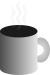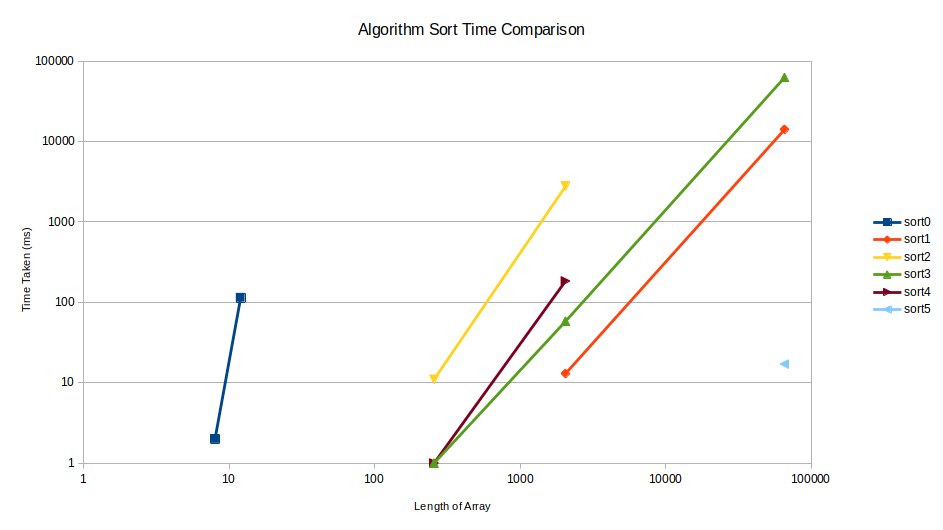#Coffee SpaceListen:

## Crazy SortingPreview Image

I recently come across this article over on HackerNews titled 'Strangest Sorting Algorithms'. I found the algorithms discussed there quite fun!

I decided to do some tests and have some fun with this. The following is a discussion regarding what I found out.

### Results

I'll start with the results, and then discuss what they actually mean.Time comparison of different algorithms (log-log)

### Algorithms

Here is a quick overview of the algorithms and the design behind them. We make the following assumptions:

1. The sorting algorithm can access any part of the array at any time, with minimal cost to access each element.
2. The sorting algorithm is aware of the length of the elements.
3. The sorting algorithm can change the elements in the given array for an in-place sort.

#### `sort0()`

So this one was more inline with the original article. For small arrays of less than 10 elements and assuming you do not want to run this sort often, it's actually not too bad at all.

```0001 /* Randomly swap, return when sorted */
0002 void sort0(int* a, int l){
0003   seed_random(0);
0004   int ordered = 0;
0005   while(ordered == 0){
0006     /* Random element */
0007     int i = rand() % l;
0008     int j;
0009     /* Random element which is not what we already selected */
0010     while((j = get_random() % l) == i);
0011     /* Swap elements */
0012     int t = a[i];
0013     a[i] = a[j];
0014     a[j] = t;
0015     /* Check if list now ordered */
0016     ordered = 1;
0017     for(int x = 1; x < l; ++x){
0018       if(a[x - 1] > a[x]){
0019         ordered = 0;
0020         break;
0021       }
0022     }
0023   }
0024 }```

A use-case for this algorithm could be:

1. Random sorting - Perhaps it is important that elements are sorted in a random order and without bias. Perhaps the movement on the element potentially gives some unfair advantage over something else represented by another element, and you need a seemingly unbiased approach to sorted the elements.
2. Limited time sort - Perhaps you want to perform a limited time search and do not want to have a biased output. With this algorithm you could make some statistical guarantees that each part of the array being sorted is equally considered.

#### `sort1()`

This algorithm is based on the classic bubble sort algorithm. It is 'blind' because it cannot end early, even if the array is already sorted. This is based on the assumption that the input array to be sorted is relatively high entropy and requires a lot of sorting, such that it's likely most operations will be required and we can save on additional checking.

```0025 /* Blind bubble sort */
0026 void sort1(int* a, int l){
0027   /* Outer pass loop */
0028   for(int pass = 0; pass < l - 1; ++pass){
0029     /* Forward iterate through list */
0030     for(int x = 1; x < l - pass; ++x){
0031       /* Compare elements and swap */
0032       if(a[x - 1] > a[x]){
0033         /* Swap elements */
0034         int t = a[x - 1];
0035         a[x - 1] = a[x];
0036         a[x] = t;
0037       }
0038     }
0039   }
0040 }```

This algorithm is of course well known and does not need to be explained. The advantage of this algorithm is it has a guaranteed run time based on the number of elements.

#### `sort2()`

This is the first of the "unreasonably effective" random-based shuffling algorithms. It finds an element out of order and swaps it randomly with some other element further up the array. It's essentially a random bubble sort.

```0041 /* Unreasonably effective random sorting */
0042 void sort2(int* a, int l){
0043   seed_random(0);
0044   int i = 0;
0045   while(i < l){
0046     /* Random element which is not what we already selected */
0047     int j = i + 1 + (get_random() % (l - (i + 1)));
0048     /* Swap elements */
0049     int t = a[i];
0050     a[i] = a[j];
0051     a[j] = t;
0052     /* Check if list now ordered */
0053     i = 0;
0054     while(++i < l){
0055       if(a[i - 1] > a[i]){
0056         --i;
0057         break;
0058       }
0059     }
0060   }
0061 }```

This is significantly faster for such simple logic.

#### `sort3()`

This is based on `sort2()`, but it looks to ensure that whichever element we swap the out-of-order element with in the later part of the list, is smaller than the element we currently have. As a result, this random shuffle performs even better.

```0062 /* Only randomly switch elements that improve the situation */
0063 void sort3(int* a, int l){
0064   seed_random(0);
0065   int i = 0;
0066   while(i < l){
0067     int j;
0068     /* Random element in higher end which is smaller than what we want to swap */
0069     while(a[j = i + 1 + (get_random() % (l - (i + 1)))] > a[i]);
0070     /* Swap elements */
0071     int t = a[i];
0072     a[i] = a[j];
0073     a[j] = t;
0074     /* Check if list now ordered */
0075     i = 0;
0076     while(++i < l){
0077       if(a[i - 1] > a[i]){
0078         --i;
0079         break;
0080       }
0081     }
0082   }
0083 }```

For small arrays $<256$ elements, this algorithm is significantly fast.

#### `sort4()`

Surprisingly, removing the randomness from `sort3()` actually slows down the algorithm!

```0084 /* Deterministic search variant of sort3() */
0085 void sort4(int* a, int l){
0086   int i = 0;
0087   while(i < l){
0088     int j = l;
0089     while(a[--j] >= a[i]);
0090     /* Swap elements */
0091     int t = a[i];
0092     a[i] = a[j];
0093     a[j] = t;
0094     /* Check if list now ordered */
0095     i = 0;
0096     while(++i < l){
0097       if(a[i - 1] > a[i]){
0098         --i;
0099         break;
0100       }
0101     }
0102   }
0103 }```

#### `sort5()`

This is a super interesting approach called "binary search trees" (BST).

```0104 struct node{ int val; struct node* left; struct node* right; };
0105
0106 void flatten(struct node* n, int* a, int* x){
0107   if(n == NULL) return;
0108   flatten(n->left, a, x);
0109   a[(*x)++] = n->val;
0110   flatten(n->right, a, x);
0111 }
0112
0113 /* Binary sort tree */
0114 void sort5(int* a, int l){
0115   struct node* nodes = malloc(sizeof(struct node) * l);
0116   int n = 0;
0117   struct node* root = &nodes[n++];
0118   root->val = a;
0119   root->left = NULL;
0120   root->right = NULL;
0121   for(int x = 1; x < l; x++){
0122     int v = a[x];
0123     struct node* parent = root;
0124     struct node* child = root;
0125     while(child != NULL){
0126       parent = child;
0127       if(a[x] < parent->val){
0128         child = parent->left;
0129       }else{
0130         child = parent->right;
0131       }
0132     }
0133     child = &nodes[n++];
0134     child->val = a[x];
0135     child->left = NULL;
0136     child->right = NULL;
0137     if(a[x] < parent->val){
0138       parent->left = child;
0139     }else{
0140       parent->right = child;
0141     }
0142   }
0143   int i = 0;
0144   flatten(root, a, &i);
0145   free(nodes);
0146 }```

1. Single pass - One massive benefit of this algorithm is that we do a single pass over the initial structure.
2. Memory heavy - We pay the cost of a value (`int`) and two pointers (`left` and `right`).
3. Limited comparisons - We can significantly reduce the number of required comparisons.
4. Unbalanced tree - It's very easy for the tree to become unbalanced and all advantage to be lost.

### Improvements

A came across some interesting ideas whilst exploring this:

1. Quicksort vs Quadsort - Quadsort is quite an interesting algorithm and performs quite well against other algorithms.
2. Tree balancing - One thing that could be done is to re-balance the binary search tree. For large arrays this would significantly reduce the depth at which we must search and place new elements.
4. ${2}^{n}$ search tree - In theory the concept of quad trees can be expanded much further. One example is an octree.
5. Sample distribution - If we pre-sample the array elements, we can throw them quite close to their goal location. We would need the ability to place elements into a non-linear structure as the distribution is unlikely to be linear.

Note: Regarding the dimensionality of ${2}^{n}$ search trees, a common method is to split via spatial dimensions. Instead we could use binning to divide the problem space into artificial spatial dimensions.

### Implementation

The source code can be found here. Here I will discuss some details regarding what I learned from this.

#### RDRAND

Intel have RDRAND, a processor-based instruction for generating random numbers. Seeing as a lot of our algorithms use random numbers, one would benefit greatly from speeding these up! To compile with RDRAND support we can use: `gcc main.c -Ofast -mrdrnd -o test`.

In the code, we enable random numbers by un-commenting the following:

`0147 //#define RDRAND // Comment this if no RDRAND support`

We generate random numbers with the following:

```0148 /* Generate random number */
0149 /* NOTE: It's possible we get bad random numbers, but we care more for speed
0150          here. */
0151 int get_random(){
0152   #ifdef RDRAND
0153     /* Source: https://www.intel.com/content/www/us/en/developer/articles/guide/intel-digital-random-number-generator-drng-software-implementation-guide.html */
0154     uint16_t rand;
0155     unsigned char ok = 0;
0156     asm volatile("rdrand %0; setc %1" : "=r" (rand), "=qm" (ok));
0157     return rand;
0158   #else
0159     /* Use C implementation */
0160     return rand();
0161   #endif
0162 }```

Ignoring the fact that the instruction cannot reliably return random numbers and needs to be checked to see whether it failed - it is also incredibly slow on my i7-6700HQ CPU. This was a complete waste of time.

#### Testing

Testing was done with the following:

```0163 void test(void (*f)(int*, int), char* name, int* t, int l, int* r, int n){
0164   int* a = malloc(l * sizeof(int));
0165   memcpy(a, t, l * sizeof(int));
0166   int64_t tb = currentTimeMillis();
0167   f(a, l);
0168   int64_t te = currentTimeMillis();
0169   printf("%i\t%s\t%s\t%li\n", l, name, cmp_arr(a, l, r, n) == 0 ? "pass" : "fail", te - tb);
0170   free(a);
0171 }```

As you can see, we only time how long it takes to process the sort and do not count the comparison or setup of the testing.

We also test each of the algorithms in turn, switching off some algorithms if the array length becomes too large (I only have so long to live):

```0172 void run_test(char* name, int* t, int l, int* r, int n){
0173   printf("Name: '%s'\n", name);
0174   if(l <= 12) test(sort0, "sort0", t, l, r, n);
0175   test(sort1, "sort1", t, l, r, n);
0176   if(l <= 2048) test(sort2, "sort2", t, l, r, n);
0177   test(sort3, "sort3", t, l, r, n);
0178   if(l <= 2048) test(sort4, "sort4", t, l, r, n);
0179   test(sort5, "sort5", t, l, r, n);
0180 }```